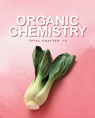Click to Chat

1800-1023-196

+91-120-4616500

CART 0

• 0

MY CART (5)

Use Coupon: CART20 and get 20% off on all online Study Material

ITEM
DETAILS
MRP
DISCOUNT
FINAL PRICE
Total Price: Rs.

There are no items in this cart.
Continue Shopping
`        In an expt on specific heat of a substance a 0.2 kg block is dropped in a calorimeter of water equivalent 0.025 kg.the final temp is 40 C.so specific heat is ?`
one year ago

```							Mass of the metal, m = 0.20 kg = 200 gInitial temperature of the metal, T1 = 150°CFinal temperature of the metal, T2 = 40°CCalorimeter has water equivalent of mass, m’= 0.025 kg = 25 gVolume of water, V = 150 cm3Mass (M) of water at temperature T = 27°C:150 × 1 = 150 gFall in the temperature of the metal:ΔT = T1 – T2 = 150 – 40 = 110°CSpecific heat of water, Cw = 4.186 J/g/°KSpecific heat of the metal = CHeat lost by the metal, θ = mCΔT … (i)Rise in the temperature of the water and calorimeter system:ΔT′’ = 40 – 27 = 13°CHeat gained by the water and calorimeter system:Δθ′′ = m1CwΔT’= (M + m′) Cw ΔT’ … (ii)Heat lost by the metal = Heat gained by the water and colorimeter systemmCΔT = (M + m’) Cw ΔT’200 × C × 110 = (150 + 25) × 4.186 × 13∴ C = (175 × 4.186 × 13) / (110 × 200)  =  0.43 Jg-1K-1If some heat is lost to the surroundings, then the value of C will be smaller than the actual value.
```
one year ago
Think You Can Provide A Better Answer ?

## Other Related Questions on Organic Chemistry

View all Questions »### Course Features

• 731 Video Lectures
• Revision Notes
• Previous Year Papers
• Mind Map
• Study Planner
• NCERT Solutions
• Discussion Forum
• Test paper with Video Solution### Course Features

• 70 Video Lectures
• Revision Notes
• Test paper with Video Solution
• Mind Map
• Study Planner
• NCERT Solutions
• Discussion Forum
• Previous Year Exam Questions